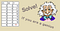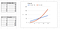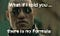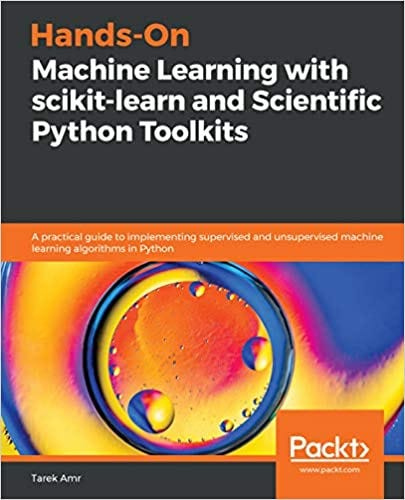# Predict the Future with Regression Analysis

## Using Scikit-Learn and a little bit of Python

The textbook definition for regression would be something like; “regression analysis is a statistical process for estimating the relationships among variables”, but seriously, who likes such a dry definitions! Let’s try another definition here then.

Do you know those annoying posts you see on Linkedin, where you are given a table with bunch of numbers, one cell is missing, and you are asked to solve it? Something like this one below?Solve if you are a genius, or just know how to use scikit-learn

Well, solving these is normally done via regression analysis. Of course, most of the ones you see on Linkedin are too easy to use anything but your kindergarten mathematics to solve, but you get the picture.

We know that the value of the last column, Y, depends on the values of X1 and X2. Take the first row, maybe it’s 14 because ‘2*5 +4 = 14’, maybe ‘3*2 + 2*4 = 14’. We don’t know what is the exact formula. So we carry on and try these formulae on the next column and see which one is the right one.

This is boring, isn’t it! What if rather than X1 and X2, we had X1 up to X100, probably no one have enough time to try all possible formulae by hand. Thus, we use regression analysis. (Actually there is one more important reason, will tell you about it later on). What we do is that we set a computer program to take the data we have, and ask it to build a model that fits our data. I.e. predict the formula that describes our data the best. We normally call the data we give to the computer to build the model, the training data.

Scikit-Learn (get it here) can help us here. We are going to use the LinearRegression module there. (I will explain to you why it is called Linear in a moment). As stated earlier, we are going to give our training data to Scikit-Learn and ask it to build the best model to fit our data. Our training data is made of rows, each row has to values of X, and a corresponding Y. For all Scikit-Learn models, you usually pass the X and Y values separately, in the format you see in the code snippet below. After training our model on the data, i.e. using the fit() method, we can simply use our trained model to predict the corresponding value of Y for X1 and X2 in the last raw.

Run this code! You got 32, right? Now you are a genius, and you can write that down instead of the red question marks.

Are you still curious, which formula is the correct formula? Typing genius_regression_model.coef_ should give you [[ 3, 2]], which means the formula is: Y = 3*X1 + 2X2. In fact, the formula is Y = 3*X1 + 2X2 + 0, that 0 part is called the intercept. The intercept can be any constant number, i.e. it is not function of X1 or X2. To see the value of the intercept you can type genius_regression_model.intercept_ which will give you a 0.0000..something, i.e. just zero, but the computer is just dumb sometimes.

## Wish life was just as simple as a linear function

You may have noticed that we used LinerRegression here. What is linear? As you have seen in the formula used, Y depended on X1 and X2, but not on something like X1², X2², X1*X2 or even X power something greater than 2, or the square root of X or such. These kind of formulae, we call them non-linear ones.Linear and Exponential Data compared

Problem is that if you try to use a linear model to model non-linear data, you will end up with a underspecified or biased model. No matter what data you feed to your model, your predictions will be wrong in the end anyway.

Most probably, you have two questions in mind now. How to tell the model we are using is a good fit or not? How can we build non-linear models in Scikit-Learn? Let me answer the second question first, because I have a philosophical surprise for you later on. Afterwards, I will answer the first question.

Time for a non-linear model using Scikit-Learn! Actually, there is some sort of hack we like to do sometimes. We can use a Liner model to build a non-Linear one. How is that? What if in the table where X takes the values of 1, 4, 7, 10 and 13, we add another column, let’s call it X2. In X2, we just put the squared values of X, i.e. 1, 16, 49, 100, 169. Now our formula will be Y = coefficient_1 * x + coefficient_2 * x2. If you have a look at the data we have now, there is a simple linear model for our data, where coefficient_1= 0 and coefficient_2 = 1, since Y equals to X², which is X2.

Rather than doing this by hand, Scikit-Learn gives us some tool called PolynomialFeatures, which we can ask to convert our values of X into all possible polynomials. Normally, in Machine Learning, we call the values of X, features, hence the name of the tool. Here comes the code:

Notice, in this example, we only have one feature, i.e. only X, no X1, X2, etc. Of course, PolynomialFeature can deal with as many features as you want. We just kept it simple here. Additionally, you can change the value of degree to expend your data into even higher degrees, x power 2, 3, 4, etc. In the end, your model during the training phase should figure out which features to ignore and which to include.

## Ain’t there other models, other that LinearRegression?

Of course, Scikit-Learn provides more complex models. Back in the early 2000’s, SVM (Support Vector Machines) was the new cool, something like Deep Learning of today. SVM can deal with non-linear data too, by using some trick called kernels. In this code, we are going to use SVM with a Polynomial kernel (i.e. non-linear) to do our regression task. We are going to use the same data as above.

If you run the SVM code snippet, you are gonna get approximate values of 36 and 21, instead of 40 and 25. Not that far away from reality, but, not that good even. SVM sucks then!?

## Life is even more complex than we thoughtIn real life, data are more noisy than you think

Real life data normally doesn’t follow a mathematical formula, and here comes the joy of Machine Learning.

Imagine you want to predict how many kilos someone will loose by tracking the steps he take every day as well as his calories intake. Number of steps and and calories intake can then be our X1 and X2. We log his weight for a while in hope to build a model where Y is the amount of kilos lost. Now, if you think about it, metabolism is a bit more complex that that. True, Y can be predicted from X1 and X2, but there are way more factors we aren’t taking into our considerations, like this person’s stress level, water intake, the weather, and many more factors I can’t even imagine. We call these factors noise, because if you try to plot Y agains X this time, it won’t be a really smooth line like the one we had here.

SVM and other models all try to deal with such imperfections of data. That’s why you cannot say, this model is always better than that one. Sometimes complex models are overkill for simple data, but they are still more effective with complex and noisy training data.

## How to tell the model we are using is a good fit or not?

Now back to your earlier question, “How to tell the model we are using is a good fit four our data or not?” Previously, we used all our data as training data. In practice, we normally split our data into two chunks. One chunk we use as our training data. I.e. we use it to train a model. The other chunk we call testing data. Once we have our model trained, we use it to predict the Y values of the testing data. Since we already know the true values of Y for the testing data anyway, we can then compare the true values to the predicted values, and decide whether our model is sound or not.

Scikit-Learn provides us with some metrics for that. For example, sklearn.metrics.mean_absolute_error and sklearn.metrics.mean_squared_error can be used to compare y_true and y_predict, mean_squared_error(y_true, y_pred), and we then can look for a model that produces minimal error.

Notice, I said minimal error, since it very unlikely to have zero error, unless there is really a formula guiding your data, which is not a usual real life scenario as I’ve just said.https://www.amazon.com/gp/product/B08BTFY8YW/

Hope you found this introduction to Scikit-Learn, Data Mining and Regression useful. If you find it useful, please check my new book, Hands-On Machine Learning with scikit-learn and Scientific Python Toolkits.

Machine Learning engineer, Data Visualisation book author, and a bunch of other things, http://www.tarekamr.com/

## More from Tarek Amr

Machine Learning engineer, Data Visualisation book author, and a bunch of other things, http://www.tarekamr.com/